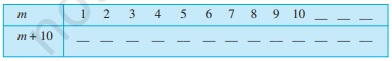(b) Complete the table and by inspection of the table
">

# (a) Complete the table and by inspection of the table find the solution to the equation $m+10=16$(b) Complete the table and by inspection of the table

To do:

We have to complete the tables and by inspection of the tables find the solutions to the equations.

Solutions:

(a) For $m + 10$, the table is represented as below:

 $m$ $m+10$ 1 $1+10=11$ 2 $2+10=12$ 3 $3+10=13$ 4 $4+10=14$ 5 $5+10=15$ 6 $6+10=16$ 7 $7+10=17$ 8 $8+10=18$ 9 $9+10=19$ 10 $10+10=20$

Therefore, from the table,

The solution to the equation $m+10=16$ is $m=6$.

(b) For $5t$, the table is represented as below:

 $t$ $5t$ 3 $5\times3=15$ 4 $5\times4=20$ 5 $5\times5=25$ 6 $5\times6=30$ 7 $5\times7=35$ 8 $5\times8=40$ 9 $5\times9=45$ 10 $5\times10=50$ 11 $5\times11=55$

Therefore, from the table,

The solution to the equation $5t=35$ is $t=7$.

(c) For $\frac{z}{3}$, the table is represented as below:

 $z$ $\frac{z}{3}$ 8 $\frac{8}{3}=2\frac{2}{3}$ 9 $\frac{9}{3}=3$ 10 $\frac{10}{3}=3\frac{1}{3}$ 11 $\frac{11}{3}=3\frac{2}{3}$ 12 $\frac{12}{3}=4$ 13 $\frac{13}{3}=4\frac{1}{3}$ 14 $\frac{14}{3}=4\frac{2}{3}$ 15 $\frac{15}{3}=5$ 16 $\frac{16}{3}=5\frac{1}{3}$

Therefore, from the table,

The solution to the equation $\frac{z}{3}=4$ is $z=12$.

(d) For $m-7$, the table is represented as below:

 $m$ $m-7$ 5 $5-7=-2$ 6 $6-7=-1$ 7 $7-7=0$ 8 $8-7=1$ 9 $9-7=2$ 10 $10-7=3$ 11 $11-7=4$ 12 $12-7=5$ 13 $13-7=6$ 14 $14-7=7$

Therefore, from the table,

The solution to the equation $m-7=3$ is $m=10$.

Updated on: 10-Oct-2022

25 Views